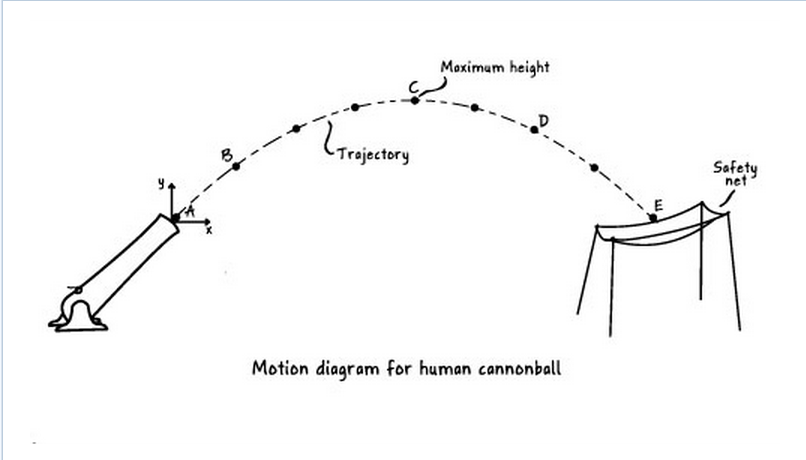# Problem: Use the motion diagram in (Figure 1)showing the trajectory of a human cannonball to answer Parts A through D. No air resistance acts on the human cannonball.Part A: How does the vertical acceleration at point A compare to the vertical acceleration at point C?a. Both accelerations are equal to free fall acceleration.b. The acceleration at A is greater than that at C.c. Both accelerations are equal to zero.d. The acceleration at A is less than that at C.

###### FREE Expert Solution

In this question, we are required to explain how verticle acceleration changes within the trajectory of a projectile.

92% (20 ratings)###### Problem Details

Use the motion diagram in (Figure 1)showing the trajectory of a human cannonball to answer Parts A through D. No air resistance acts on the human cannonball.

Part A: How does the vertical acceleration at point A compare to the vertical acceleration at point C?

 a. Both accelerations are equal to free fall acceleration. b. The acceleration at A is greater than that at C. c. Both accelerations are equal to zero. d. The acceleration at A is less than that at C.